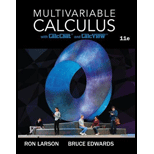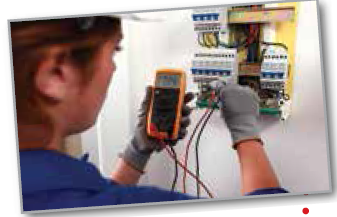# Electrical Circuits In Exercises 29 and 30, use the electrical circuit differentialequation d 2 q d t 2 + ( R L ) d q d t + ( 1 L C ) q = ( 1 L ) E ( t ) where R is theresistance (in ohms), C is the capacitance(in farads), L is theinductance (in henrys), E ( t )is the electromotiveforce (in volts), and q is the charge on thecapacitor (in coulombs).Find the charge q asa function of time t for the electrical circuit described. Assume that q ( 0 ) = 0 and q ' ( 0 ) = 0 . R = 20 , C = 0.02 , L = 2 , E ( t ) = 12 sin 5 t### Multivariable Calculus

11th Edition
Ron Larson + 1 other
Publisher: Cengage Learning
ISBN: 9781337275378

#### Solutions

Chapter
Section### Multivariable Calculus

11th Edition
Ron Larson + 1 other
Publisher: Cengage Learning
ISBN: 9781337275378
Chapter 16.3, Problem 29E
Textbook Problem
6 views

## Electrical CircuitsIn Exercises 29 and 30, use the electrical circuit differentialequation d 2 q d t 2 + ( R L ) d q d t + ( 1 L C ) q = ( 1 L ) E ( t ) where R is theresistance (in ohms),C is the capacitance(in farads), L is theinductance (in henrys),E(t)is the electromotiveforce (in volts), and q is the charge on thecapacitor (in coulombs).Find the charge q asa function of time t for the electrical circuit described. Assume that q ( 0 ) = 0 and q ' ( 0 ) = 0 .R = 20 , C = 0.02 , L = 2 , E ( t ) = 12 sin 5 t

To determine

To calculate: The charge q as a function of time t for the electrical circuit describedfor the differential equation is d2qdt2+(RL)dqdt+(1LC)q=(1L)E(t) where, R=20,C=0.02,L=2,E(t)=12sin5t.

### Explanation of Solution

Given information:

The given differential equation is d2qdt2+(RL)dqdt+(1LC)q=(1L)E(t) where R=20,C=0.02,L=2,E(t)=12sin5t and assume that q(0)=0and q'(0)=0.

Concept Used:

If yp is the particular solution of the differential equation y''+ay'+by=F(x) and yh is the general solution, then y=yh+yp is the general solution of the non homogeneous equation.

Calculation:

Consider the given differential equation is d2qdt2+(RL)dqdt+(1LC)q=(1LC)E(t).

The coefficients of the differential terms are,

RL=202=101LC=12×0.02=251L=12=0.5

Now putting these in the differential equation

d2qdt2+10dqdt+25q=0.5×12sin5td2qdt2+10dqdt+25q=6sin5t

The characteristics equation is m2+10m+25=0.

First, we have to find the solution of the characteristics equation m2+10m+25=0.

m2+10m+25=0(m+5)2=0m=5

Therefore,

qh=(C1+tC2)e5t

Let qp be generalized form of 6sin5t

### Still sussing out bartleby?

Check out a sample textbook solution.

See a sample solution

#### The Solution to Your Study Problems

Bartleby provides explanations to thousands of textbook problems written by our experts, many with advanced degrees!

Get Started

Find more solutions based on key concepts
In Exercises 6372, evaluate the expression. 67. 32+33

Applied Calculus for the Managerial, Life, and Social Sciences: A Brief Approach

For , an upper bound estimate for s − s6 (where s6 is the sixth the series does not converge

Study Guide for Stewart's Single Variable Calculus: Early Transcendentals, 8th

Find y and y. y=xlnx

Single Variable Calculus: Early Transcendentals, Volume I

Using Quadratic Regression In Exercises S-13 through S-20, use regression to find a quadratic model for the giv...

Functions and Change: A Modeling Approach to College Algebra (MindTap Course List)

11. Competition in the personal computer market is intense. A sample of 450 purchases showed 202 Brand A comput...

Modern Business Statistics with Microsoft Office Excel (with XLSTAT Education Edition Printed Access Card) (MindTap Course List)

In Problems 3 and 4 use Eulers method to obtain a four-decimal approximation of the indicated value. First use ...

A First Course in Differential Equations with Modeling Applications (MindTap Course List)